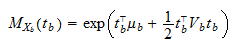StatLect

Multivariate normal distribution - Partitioned vectors

Letbe amultivariate normal random vector with meanand covariance matrix. In this lecture we present some useful facts about partitionings of, that is, about subdivisions ofinto two sub-vectorsandsuch thatwhereandhave dimensionsandrespectively and.Notation

In what follows, we will denote by:

•the mean of;

•the mean of;

•the covariance matrix of;

•the covariance matrix of;

•the cross-covariance betweenand.

Given this notation, it follows thatandNormality of the sub-vectors

The following proposition states a first (trivial) fact about the two sub-vectors.

Proposition Bothandhave a multivariate normal distribution, i.e.,Proof

The random vectorcan be written as a linear transformation of:whereis amatrix whose entries are either zero or one. Thus,has a multivariate normal distribution, because it is a linear transformation of the multivariate normal random vectorand multivariate normality is preserved by linear transformations (see the lecture entitled Linear combinations of normal random variables). By the same token, alsohas a multivariate normal distribution, because it can be written as a linear transformation of:whereis amatrix whose entries are either zero or one.

This, of course, implies that any sub-vector ofis multivariate normal whenis multivariate normal.

Independence of the sub-vectors

The following proposition states a necessary and sufficient condition for the independence of the two sub-vectors.

Propositionandare independent if and only if.

Proofandare independent if and only if their joint moment generating function is equal to the product of their individual moment generating functions (see the lecture entitled Joint moment generating function). Sinceis multivariate normal, its joint moment generating function isThe joint moment generating function ofisThe joint moment generating function ofand, which is just the joint moment generating function of, isfrom which it is obvious thatif and only if.

The book

Most of the learning materials found on this website are now available in a traditional textbook format.

Glossary entries
Share